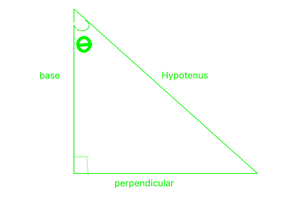Prove tan2 θ – (1/cos2 θ) + 1 = 0

• Last Updated : 06 Oct, 2021

It is basically a study of the properties of the triangle and trigonometric function and its application in various cases. It helps find the angles and missing sides of the triangle with the help of trigonometric ratios. Generally, some angle’s values are known from which we get the value of other angles. The commonly known angle angles are 0°, 30°, 45°, 60°, and 90°.

Trigonometry is known for its identities. The trigonometric identities are commonly used for rewriting trigonometrical expressions to simplify an expression, to get a more useful sort of an expression, or to unravel an equation. Problems in which a one-dimensional plane is used are done with the help of plane trigonometry. Applications to similar problems in additional than one plane of three-dimensional space are considered in trigonometry.

Trigonometric Ratios

Trigonometric ratios are the ratios between sides of a right-angled triangle. These ratios are given by the following trigonometric functions of the known angle, where perpendicular, base, and hypotenuse refer to the lengths of the sides in the below figure,The hypotenuse is the side opposite to the right angle, it is the largest side in the triangle. The base is the side that contains the angle. Perpendicular is the side opposite to the given angle.

The six important trigonometric functions (trigonometric ratios) are calculated using the below formulas and considering the above figure. It is necessary to get knowledge about the sides of the right triangle because it defines the set of important trigonometric functions.

• The sine of θ is written as sinθ and defined as the ratio sinθ = perpendicular/hypotenuse
• The cosine of θ is written as cosθ and defined as the ratio cosθ = base/hypotenuse
• The tangent of θ is written as tanθ and defined as the ratio tanθ = perpendicular/base = sinθ/cosθ

Note The reciprocals of sine, cosine, and tangents also have names: they are cosecant, secant, and cotangent.

• The cosecant of θ is written as cosecθ and defined as cosecθ = 1/sinθ
• The secant of θ is written as secθ and defined as secθ = 1/cosθ
• The cotangent of θ is written as cotθ and defined as cotθ = 1/tanθ

Trigonometric ratio table

There are three Pythagorean Identities,

1. sin2 θ + cos2θ = 1
2. tan2θ + 1 = sec2θ
3. cot2 θ + 1 = cosec2 θ

Prove: tan2 θ – (1/cos2 θ) + 1 = 0

Solution:

To prove – tan2θ – (1/cos2θ) + 1 = 0

It is known,

⇒ 1/cosθ = secθ

So the equation becomes,

⇒ tan2θ – sec2θ + 1 = 0

From the Pythagorean identity, tan2θ + 1 = sec2θ

So the equation becomes,

⇒ sec2θ – sec2θ which is clearly equal to 0

⇒ tan2θ – (1/cos2θ) + 1 = 0

Hence Proved

Sample Problems

Question 1: Find the value of sin2x – cos2x in terms of sinx

Solution:

⇒ sin2x + cos2x = 1 [From pythagorean identity]

So,

cos2x = 1 – sin2

Putting this,

sin2x – (1 – sin2x) which is equal to

2sin2x – 1

Question 2: Find the value of 12tan2x – 12sec2x + 12

Solution:

⇒ 12tan2x – 12sec2x + 12 ⇢ 12(tan2x – sec2x) + 1

It is known, sec2x – tan2x = 1 [from pythagorean identity]

So, the equation becomes 12(-1) + 12 = 0

12tan2x – 12sec2x + 12 = 0

Question 3: If tanx = 3 find the value of sec2x + cosec2x

Solution:

⇒ It is known, sec2x = 1 + tan2x [from pythagorean identity]

cosec2x = 1 + cot2x [from pythagorean identity]

Also, cotx = 1/tanx

Equation becomes 2 + tan2x + cot2x

Putting the values,

2 + 9 + 1/9 = 100/9

sec2x + cosec2x, when tanx = 3 ⇒ 100/9

My Personal Notes arrow_drop_up